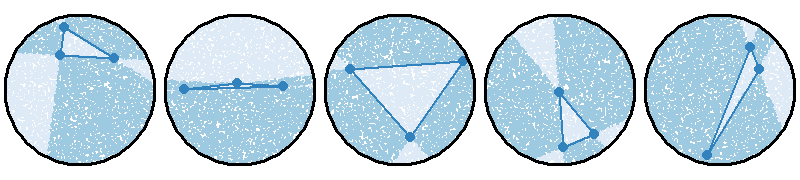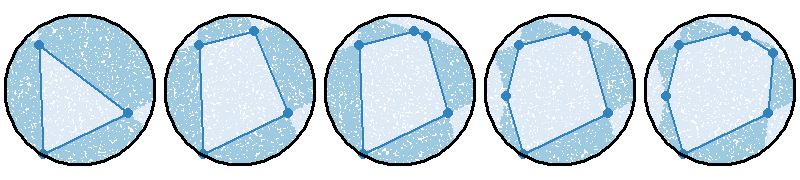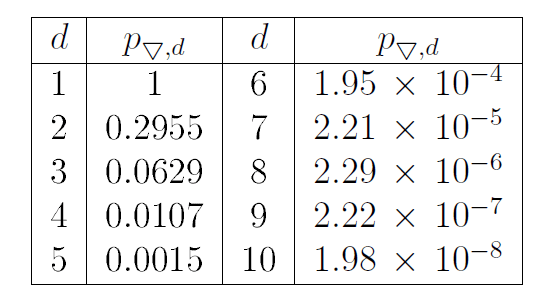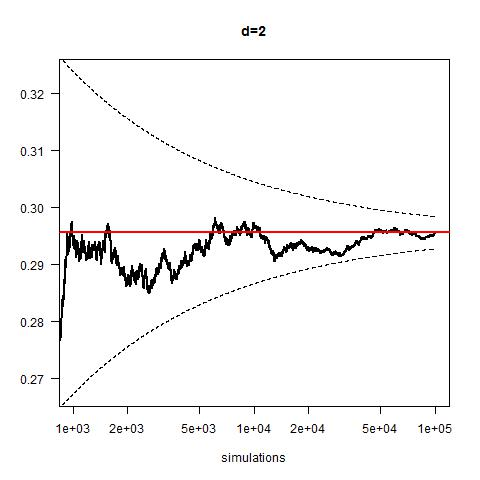# Sylvester’s convex hull problem in R

What is the probability that d+2 random points in d-dimensional space form a convex body? Investigating an old problem using modern methods.James Joseph Sylvester was born just over 200 years ago on 3 September 1814 in London and was educated at Cambridge. However, as a Jew, he was awarded his degree only in 1872 when Oxford and Cambridge Universities abolished the theological tests for graduates. In 1838 he became professor of Natural Philosophy at University College London (the first English university to admit students regardless of race, class or religion, and also the first to admit men and women on an equal basis) where he had been, briefly, an undergraduate ten years earlier, and three years later was appointed to the chair of mathematics at the University of Virginia in Charlottesville, a post he held for a few months. In 1854 he was appointed to the chair of mathematics at the Royal Military Academy at Woolwich until his retirement, under military rules, at age 55. This was followed by a fruitful period as a mathematician. In 1877, Sylvester went back to the United States as professor at Johns Hopkins University, and, aged 68, in 1883 was appointed Savilian professor of geometry at Oxford. He retired in 1892 and died in London on 15th March 1897. The Royal Society instituted the Sylvester Medal in 1901 for the encouragement of mathematical research.

## The convex hull problem

Sylvester made many important contributions to mathematics, notably in linear algebra and geometric probability. In this article we look at a problem Sylvester first proposed in 1864 in the Educational Times of London:

Show that the chance of four points forming the apices of a re-entrant quadrilateral is 1/4 if they be taken at random in an indefinite plane.A convex shape is one in which it is possible to connect any two points within the shape with a straight line that doesn’t leave the shape. The arrow-head on the right is concave, since the red line passes outside of the shape.

In other words, he called for a proof that four, connected, randomly drawn, points on an infinite plane resulted in a re-entrant (concave) quadrilateral 1/4 of the time.
This problem has no solution, as there is no natural probability measure on an infinite plane. The following year Sylvester modified the question thus:

Let $K$ be a convex body in the plane and choose four random, independent and uniform points from $K$. What is the probability that they form the vertices of a re-entrant quadrilateral?

In contemporary terminology it can be phrased as “what is the probability that the convex hull of four randomly distributed points on a region $K$ of the plane is a triangle?” (A set in Euclidean space is convex if for every pair of points in the set the straight line segment joining the pair is also within the set; the convex hull of a set $P$ of points in Euclidean space is the smallest convex set that contains $P$.)

In general, if $K$ is any $d$-dimensional convex region the problem requires $d + 2$ independent, uniformly distributed points on $K$ and there are only two possibilities: these points’ convex hull has either $d + 2$ or $d + 1$ vertices.The two possible configurations of five points within the unit sphere. The four-point base of the pyramid appears in black on the left.

It is easy to imagine how convex hulls with $d + 1$ vertices, ie tetrahedra in 3 dimensions, would become rarer in higher dimensions. For example, it might be useful to think about the problem as a constraint problem with each added dimension requiring one additional constraint. In the two dimensional case we start with a random triangle and look at what proportion of the space could the point of a convex quadrilateral be formed.Five simulations of Sylvester’s problem in two dimensions, seen as a constraint problem. The dark areas correspond to points where the fourth point could be placed to form a convex quadrilateral.

## In higher dimensions

As the number of dimensions becomes higher, the shape formed from the increased number of points becomes more complex. These higher dimensions increase the constraints on the feasible vertices, reducing the probability that the shape formed is convex. Adding points, even while adding additional dimensions, will increase the number of constraints in at least one $2-d$ cross section. Adding additional points to form an increasingly complex parallelogram in a $2-d$ space results in increasingly scarce feasible areas. The figure below shows simulations in higher dimensions, with the number of points corresponding to the number of dimensions.Five simulations of Sylvester’s problem, seen as a constraint problem, in higher dimensions.

It is known that the shape of $K$ determines the probabilities of such events, and that among simple planar regions, the probability of interest reaches its maximum value of 1/3 if $K$ is a triangle and its minimum, $35/(12 \pi^2)$ if $K$ is an ellipse, and that the extremality property of ellipses holds in higher dimensions. In a remarkable paper published in 1969, John Kingman showed, purely based on combinatorial methods, that the probability that the convex hull of $d + 2$ randomly chosen points in the $d$-dimensional unit ball $B^{(d)}$, has $d + 1$ vertices, ie tetrahedra in $B^{(3)}$ and simplices in $d \ge 4$, is:

$$p_{\bigtriangledown, d} = \frac{(d + 2) \, \binom{d+1}{(d+1)\,/\,2}^{d+1}}{2^d\, \binom{(d+1)^2}{(d+1)^2\,/\,2}},$$

where the binomial coefficients are generally defined in terms of the Gamma function, ie

$$\binom{a}{b} = \frac{\Gamma\,(a+1)}{\Gamma\,(b+1)\ \Gamma\,(a-b+1)}.$$
Since $\Gamma\,(1/2)= \sqrt{\pi}$ we have
$$p_{\bigtriangledown, 2} = 35\,/\,(12 \pi^2 ) \approx 0.2955,$$
and
$$p_{\bigtriangledown, 3} = 9\,/\,143 \approx 0.0629.$$For each dimension $d$, the probability that $d+2$ points has a convex hull with $d+1$ vertices.

Estimating the probabilities in the table above empirically can be easily programmed in the statistical language R. The solution is simple: generate $d + 2$ uniformly distributed points within $B^{(d)}$, compute their convex hull and count how many times it has $d + 1$ vertices out of $M$ simulations. An algorithm due to Marsaglia efficiently generates uniformly distributed points within $B^{(d)}$.

We first simulate a vector of $d$ independent points each from a standard normal distribution, and scale them dividing them by their modulus, ie the square root of their sum of squares. These coordinates correspond to points on the surface of $B^{(d)}$, which are points within $B^{(d-1)}$. To obtain points within $B^{(d)}$ we simply divide them by the $d$th root of uniformly distributed radii between 0 and 1. We then use a function in R called convhulln to generate the $d$-dimensional convex hull.

As higher dimensions and more points are introduced the problem becomes increasingly computationally demanding due to the need to calculate whether or not the points form a convex hull. It is at this point that we become grateful that someone has done the difficult maths to identify the exact probability solution generally for any dimension. For instance if one wanted to approximate the probability of observing a convex hull in an 8-dimensional space with nine vertices, the simulation would only reveal one positive result for around every 50 million negatives. Many more than a single positive result are necessary in order to attempt any claim at identification. As dimensionality increases even further, any scale of computational resources becomes exhausted. For example, the figures below show the results for $d = 2$ and $d =5$ for $10^5$ and $10^6$ simulations, respectively with 95% confidence limits.Monte Carlo approximation for Sylvester’s problem in 2 dimensions, with $10^{5}$ simulations.Monte Carlo approximation for Sylvester’s problem in 5 dimensions, with $10^{6}$ simulations.

Like many classic problems in mathematics, eg Hardy and Ramanujan’s Taxi number or the four-colour problem, Sylvester’s problem, its generalisations and solutions can be explained in a couple of minutes with no reference to the methods required to solve it. Finding Monte Carlo approximations to its probabilities is an interesting challenge; we first read about it in Brian Ripley’s book on Stochastic Simulation where the author claims to have found a satisfactory approximation for $d=2$ in “half an hour, using a VAX 11/782 (including programming)”.

Writing a few R functions to provide a general solution took us a bit more time but it was fun!